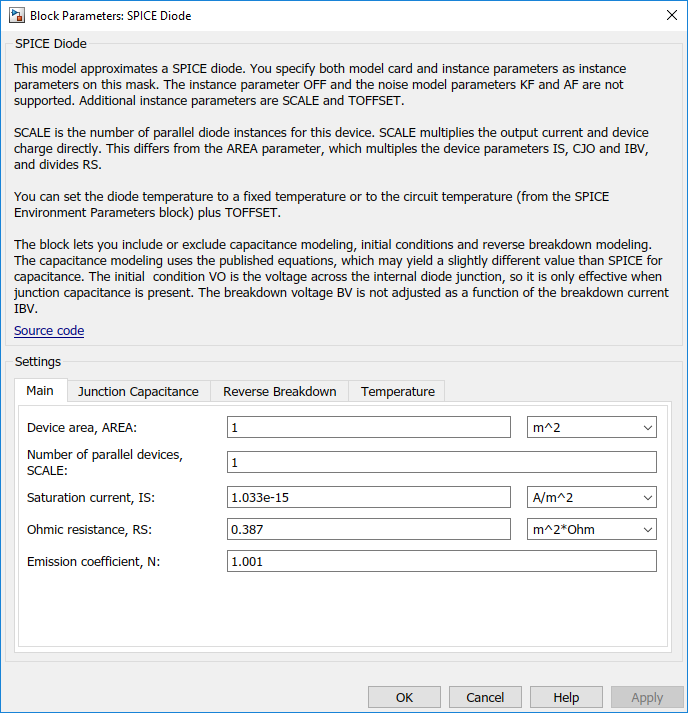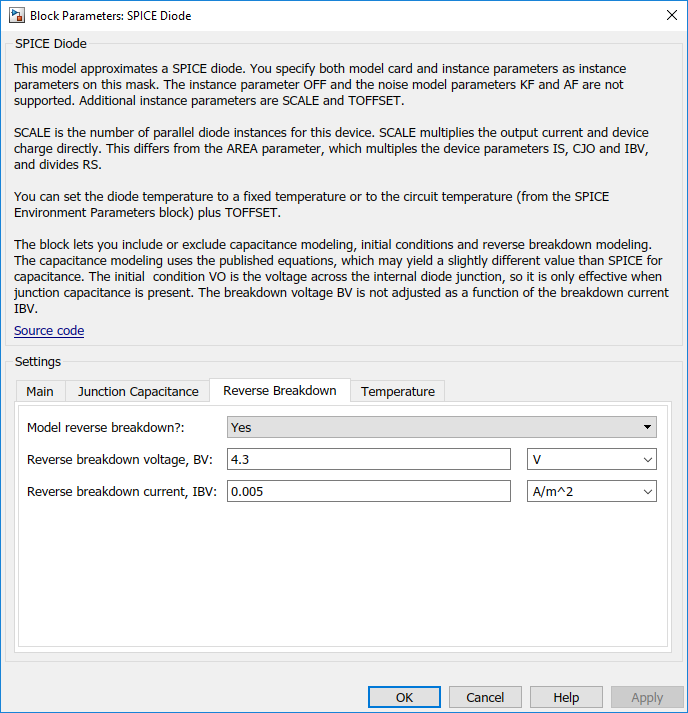## Parameterize an Exponential Diode from SPICE Netlist

If a datasheet does not provide all of the data required by the component model, another source is a SPICE netlist for the component. Components are defined by a particular type of SPICE netlist called a subcircuit. The subcircuit defines the coefficients for the defining equations. Most component manufacturers make subcircuits available on their websites. The format is ASCII, and you can directly read off the parameters. The BZX384-B4V3 subcircuit can be obtained from Philips Semiconductors.

The subcircuit data can be used to parameterize the Simscape™ Electrical™ Diode block either in conjunction with the datasheet, or on its own. For example, the Ohmic resistance is defined in the subcircuit as RS = 0.387, thus providing the missing piece of information in Example 2.

An alternative workflow is to use the Simscape Electrical Additional Components/SPICE Semiconductors sublibrary. The SPICE Diode block in this sublibrary can be directly parameterized from the subcircuit by setting:

• Saturation current, IS to `1.033e-15`

• Ohmic resistance, RS to `0.387`

• Emission coefficient, N to `1.001`

• Zero-bias junction capacitance, CJO to `2.715e-10`

• Junction potential, VJ to `0.7721`

• Grading coefficient, M to `0.3557`

• Capacitance coefficient, FC to `0.5`

• Reverse breakdown current, IBV to `0.005`

• Reverse breakdown voltage, BV to `4.3`

Note that where there is a one-to-one correspondence between subcircuit parameters and datasheet values, the numbers often differ. One reason for this is that datasheet values are sometimes given for maximum values, whereas subcircuit values are normally for nominal values. In this example, the CJO value of 271.5 pF differs from the datasheet capacitance of 450 pF at zero bias for this reason.